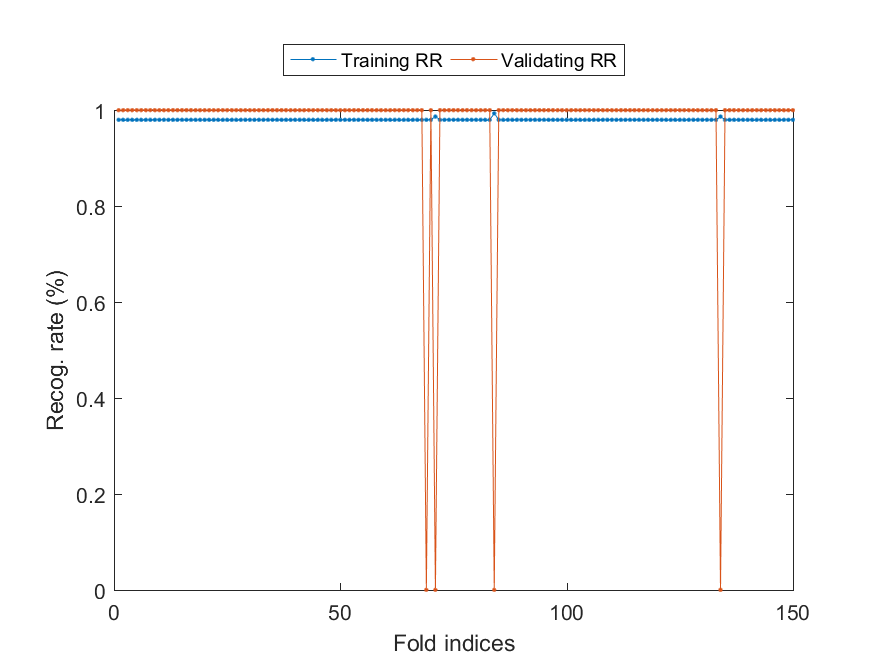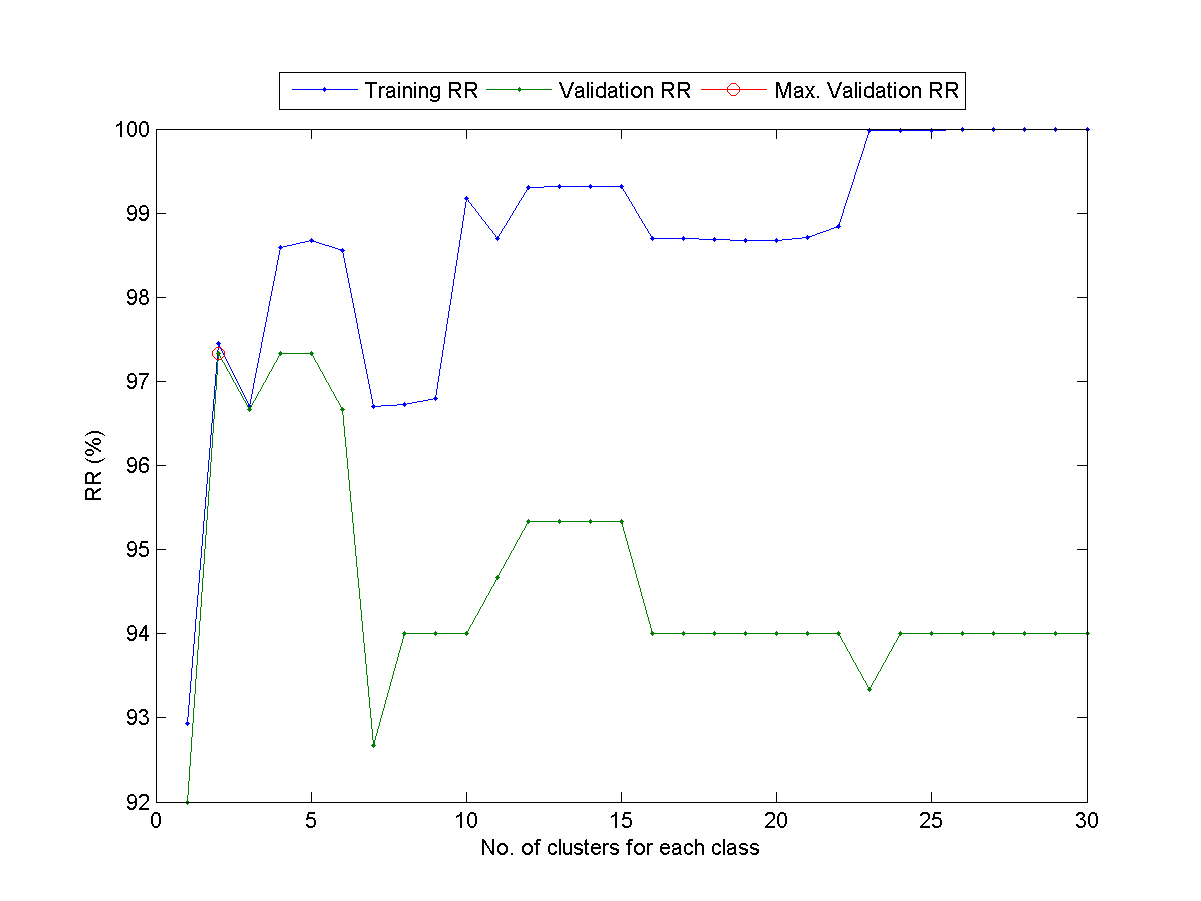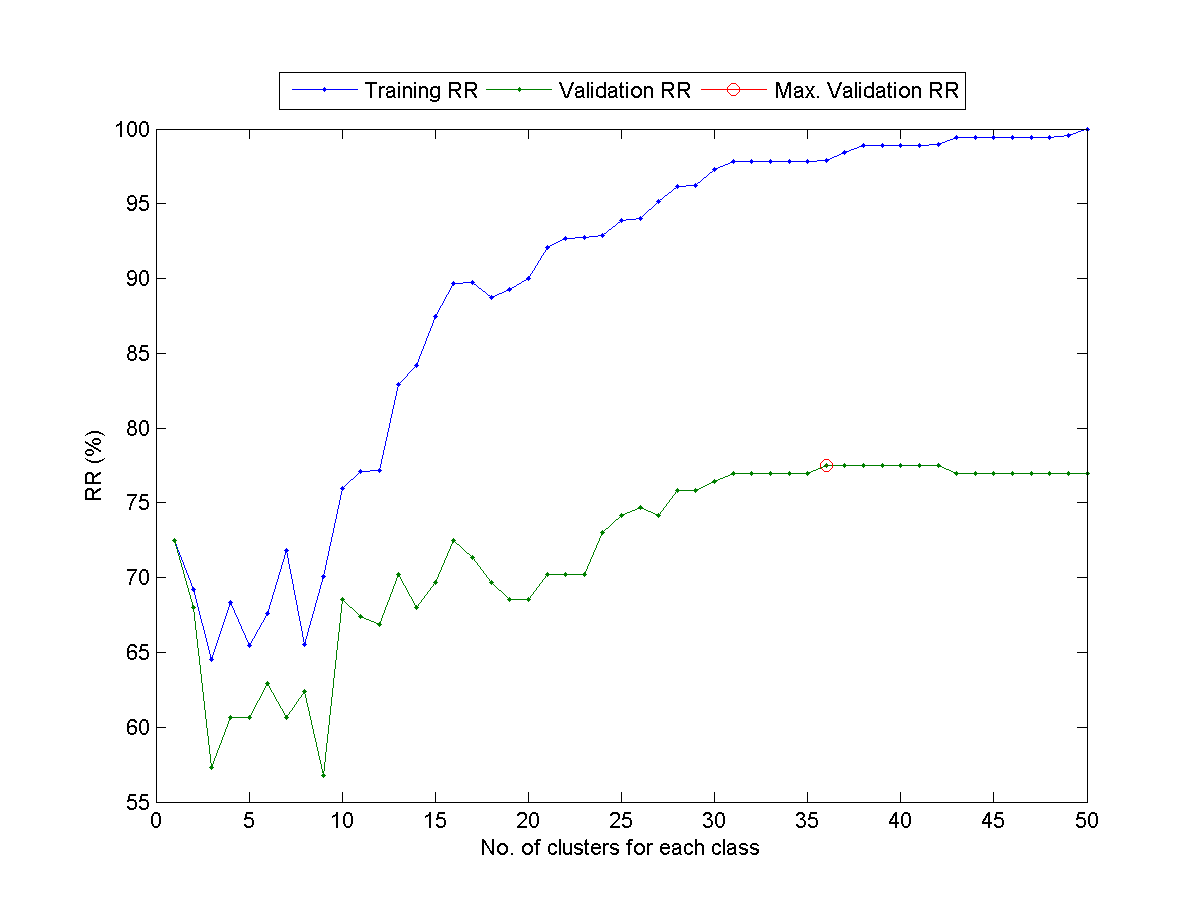Chapter 6: Exercises

1. (*) Cross validation: Explain the following methods for performance evaluation of classifiers:
1. Hold-out test
2. Two-way hold-out test
3. m-fold cross validation
4. Leave-one-out cross validation
2. (**) About m-fold cross validation:
1. Explain the basic principle of m-fold cross validation.
2. What are the strength and drawback of it when compared with one-sided holdout test?
3. (**) About leave-one-out cross validation:
1. Explain the basic principle of leave-one-out cross validation.
2. What are the strength and drawback of it when compared with m-fold cross validation (where m is less than the size of the training dataset)?
4. (**) LOO CV for QC: What is your strategy to save computation as much as possible if you want to compute LOO cross validation for
1. QC
2. NBC
5. (**) 5-fold cross validation on IRIS using 1-NNC: Write a script to perform 5-fold cross validation on the Iris dataset using 1-NNC.
1. What is the outside-test recognition for each fold of the 5-fold cross validation?
2. What is the overall outside-test recognition rate?
(Hint: You can use the function cvDataGen.m to divide the dataset into 5 parts, each with similar data count for a class.)
6. (**) 5-fold cross validation on WINE using 1-NNC: Repeat the previous exercise by using the WINE dataset.
7. (**) Speeding up LOOCV of QC: By using perfCv.m, it is pretty easy to compute the training and validation accuracy via LOOCV (leave-one-out cross validation). Here is an example which performs LOOCV on the IRIS dataset:

Example 1: perfCv4qc02.mDS=prData('iris'); showPlot=1; foldNum=inf; % For leave-one-out cross validation classifier='qc'; tic [vRrAll, tRrAll]=perfCv(DS, classifier, [], foldNum, showPlot); fprintf('time=%g sec\n', toc); fprintf('Training RR=%.2f%%, Validating RR=%.2f%%\n', tRrAll*100, vRrAll*100); time=0.213905 sec Training RR=98.00%, Validating RR=97.33%However, perfCv.m performs LOOCV by viewing the model construction as a black box. To speed up LOOCV, we can take advantage of our insight into the model construction and try to compute a common part that can be used repeatedly. For the model construction without the k-th I/O pairs, we can simply perform a quick update to obtain the new models. You mission in this exercise is to write a function myPerfCv.m that can perform fast LOOCV using QC, with the following format:
[vRr, tRr]=myPerfCv(DS);
where vRr and tRr are the validation and training recognite rates, respectively.
Hint: You should compute common parts first
• $\mu_{all}=\frac{1}{n} \sum_{i=1}^n x_i$
• $\Sigma_{all} = \frac{1}{n} \sum_{i=1}^n (x_i-\mu_{all})(x_i-\mu_{all})^T$
Then you can update the parameters when an entry $x_k$ is removed from the dataset.
8. (**) Speeding up LOOCV of NBC: Repeat the previous exercise by using NBC (naive Bayes classifier).
9. (**) Determine the best number of centers for VQ-based 1-NNC: Write a script to determine the best number of centers for VQ-based 1-NNC using LOOCV. Specifically, you should follow the steps:
1. For a given dataset, you should be able to run perfCv.m to return the LOO validation and training RRs for a given value of c (the number of the centers for VQ-based 1-NNC). Here are two examples for your reference:
2. Increase the value of c and and plot the validation RR (vRr) and the training RR (tRr) as two curves with respect to c.
3. In fact, the value of c represents the complexity of the classifier. Can you observe the phenomenon that the training RR goes up with c all the way, while the validation RR goes up initially and then fall off eventually? Please plot the curves and show the plot to TA. At which value of c the validation RR achieves its maximum?
Here is the result when applied to IRIS dataset:This is the resuslt for WINE dataset:10. (*) Train and evaluate a classifier: Write a function myTrainTest.m to train and evaluate a classifier based on given training and test datasets. The usage of the function is
[trainRr, testRr]=myTrainTest(ds4train, ds4test, classifier)
where
• ds4train: dataset for training
• ds4test: dataset for testing
• classifier: 'qc' for quadratic classifier, 'nbc' for naive Bayes classifier
• trainRR: recognition rate of training
• testRr: recognition rate of testing
(You need to use the Machine Learning Toolbox directly. You can try "help nbcTrain" and "help nbcEval" to see how to train and test NBC classifier, etc.)

Test script:

Example 2: myTrainTestTest.m% Test script for myTrainTest.m % You need to change the following line to add Machine Learning Toolbox to your MATLAB search path addpath d:/users/jang/matlab/toolbox/machineLearning dsName='iris'; classifier='nbc'; [ds4train, ds4test]=prData(dsName); [trainRr, testRr]=myTrainTest(ds4train, ds4test, classifier); fprintf('dsName=%s, classifier=%s, trainRr=%f, testRr=%f\n', dsName, classifier, trainRr, testRr); dsName='iris'; classifier='qc'; [ds4train, ds4test]=prData(dsName); [trainRr, testRr]=myTrainTest(ds4train, ds4test, classifier); fprintf('dsName=%s, classifier=%s, trainRr=%f, testRr=%f\n', dsName, classifier, trainRr, testRr); dsName='wine'; classifier='nbc'; [ds4train, ds4test]=prData(dsName); [trainRr, testRr]=myTrainTest(ds4train, ds4test, classifier); fprintf('dsName=%s, classifier=%s, trainRr=%f, testRr=%f\n', dsName, classifier, trainRr, testRr); dsName='wine'; classifier='qc'; [ds4train, ds4test]=prData(dsName); [trainRr, testRr]=myTrainTest(ds4train, ds4test, classifier); fprintf('dsName=%s, classifier=%s, trainRr=%f, testRr=%f\n', dsName, classifier, trainRr, testRr); dsName=iris, classifier=nbc, trainRr=0.960000, testRr=0.946667 dsName=iris, classifier=qc, trainRr=0.973333, testRr=0.960000 dsName=wine, classifier=nbc, trainRr=0.977528, testRr=0.988764 dsName=wine, classifier=qc, trainRr=1.000000, testRr=1.000000

Data Clustering and Pattern Recognition (資料分群與樣式辨認)